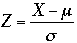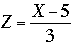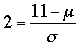SEARCH HOMEMath Central Quandaries & QueriesQuestion from Barb, a student: I am having trouble finding information on a z score and the conversion to the number of standard deviations a z value can be away from the mean. What exactly does that mean and what am I looking for? Help Please.Hi Barb,

In probability and statistics the letter Z is used for the random variable which has the standard normal distribution, that is the normal distribution with mean 0 and standard deviation 1. Many times we have a random variable X from the normal distribution with some mean μ and standard deviation σ, and we convert it to the standard normal random variable Z usingthe so called Z-score.

Lets look at a specific example. Suppose you have a normal distribution with mean μ = 5 and standard deviation σ = 3. If the random variable that satisfies this distribution is X thenSuppose you now take a measurement from this distribution and obtain a value X = 11. This gives Z = 2 or said using symbolsMultiplying both sides by σ gives

11 - μ = 2 σ

which says that 11 is 2 standard deviations more than the mean. Suppose we take another measurement X and this time get Z = -1.5. Then

X - μ = -1.5 σ

and hence X is one and a half standard deviations less than the mean.

Hence the Z-score tell us how far X is from the mean using the units of standard deviation.

I hope this helps,
HarleyMath Central is supported by the University of Regina and The Pacific Institute for the Mathematical Sciences.